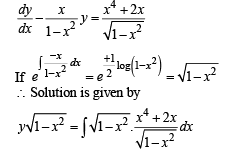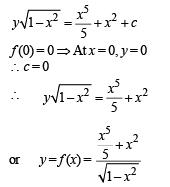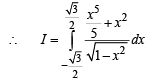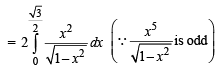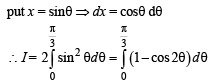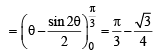Courses

# Test: Single Correct MCQs: Differential Equations | JEE Advanced

## 9 Questions MCQ Test Mathematics For JEE | Test: Single Correct MCQs: Differential Equations | JEE Advanced

Description
This mock test of Test: Single Correct MCQs: Differential Equations | JEE Advanced for JEE helps you for every JEE entrance exam. This contains 9 Multiple Choice Questions for JEE Test: Single Correct MCQs: Differential Equations | JEE Advanced (mcq) to study with solutions a complete question bank. The solved questions answers in this Test: Single Correct MCQs: Differential Equations | JEE Advanced quiz give you a good mix of easy questions and tough questions. JEE students definitely take this Test: Single Correct MCQs: Differential Equations | JEE Advanced exercise for a better result in the exam. You can find other Test: Single Correct MCQs: Differential Equations | JEE Advanced extra questions, long questions & short questions for JEE on EduRev as well by searching above.
QUESTION: 1

### A solution of the differential equationSolution: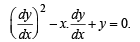By actual verification we find that the choice (c), i.e. y = 2x-4 satisfies the given differential equation.

QUESTION: 2

### If x2 + y2 = 1, then

Solution:

Given x2 + y2 = 1. Differen tiat ing w.r.t . x , we get 2x + 2yy ' = 0 or x + yy '= 0. Again differentiating w.r.t. x, we get 1 + y ' y '+ yy " = 0 or 1 + (y')2 + yy" = 0

QUESTION: 3

### If y(t) is a solution of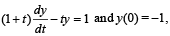then y(1) is equal to

Solution:

The given differential equation is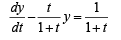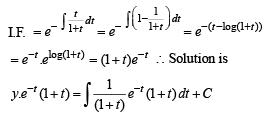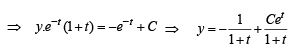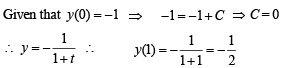QUESTION: 4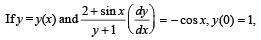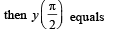Solution: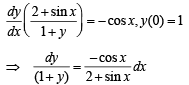Integrating both sides
⇒ ln(1 + y) = - ln(2 + sin x)+C
Put x = 0 andy=1 ⇒ ln(2) = - ln 2+ C ⇒ C = ln4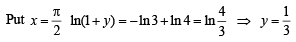QUESTION: 5

If y = y(x) and it follows the relation x cos y + y cos x = π then y" (0) =

Solution: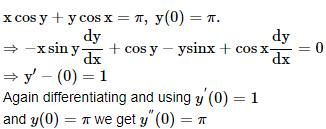QUESTION: 6

The solution of primitive integral equation (x2 + y2) dy = xy dx is y = y(x). If y (1) = 1 and (x0) = e, then x0 is equal to

Solution:

The given D.E. is (x2 + y2)dy = xy dx s.t. y (1) = 1 and y(x0) = e

The given eqn can be written as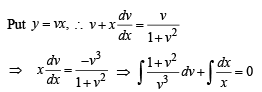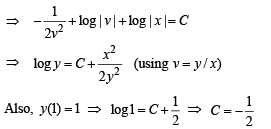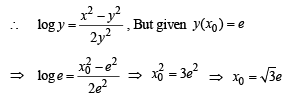QUESTION: 7

For th e primitive in tegral equation ydx + y2dy = x dy; x ∈ R, y > 0, y = y(x), y(1) = 1, then y(–3) is

Solution:

The given eqn is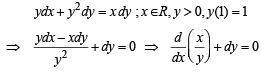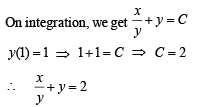Now to find y(-3), puttin g x =-3 in above eqn, we get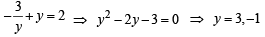But given that y > 0 , ∴ y = 3

QUESTION: 8

The differential equation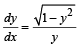determines a family of circles with

Solution: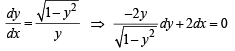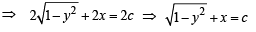⇒ ( x - c)2 + y2 = 1

which is a circle of fixed radius 1 and variable centre (c, 0) lying on x-axis.

QUESTION: 9

The function y = f (x) is the solution of the differential equation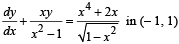satisfying f (0) = 0. Then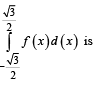Solution:

Given D.E. can be written as